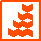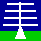HOME

ANNOUNCEMENTS

CONTACTS

DATA

INSTRUMENTS

MISCELLANEOUS

PLOTS

PUBLICATIONS

SCIENCE

WIND-PROFILER
PRINCIPLES

THE NERC MST RADAR FACILITY AT ABERYSTWYTH
FILE FORMAT FOR LEGACY MST RADAR DOPPLER SPECTRA (VERBOSE DESCRIPTION)File contents
These files contain MST Radar return Doppler spectra, for several cycles of observation. Each dwell may contain data for ST-mode observations (which cover the approximate altitude range 2 - 20 km) or for M-mode observations (which cover the approximate altitude range 58 - 94 km) or for both together. An overview of the radar measurement technique and of the meanings of the data products is given here.

Availability
MST radar spectral data are only available in this format up to 6th February 2007. Data for later dates are available in netCDF files.

File naming convention:
dsYYMMDD_hhmm.ddYYis a 2-digit year [00 - 99] MM is a 2-digit month [01 - 12] DD is a 2-digit day [01 - 31] hh is a 2-digit hour [00 - 23] DD is a 2-digit minute [00 - 59] dd is the integer number of minutes covered by the file

Archiving convention: YYYY/mon

File availability
Doppler spectra data are available from 1990 onwards.

File format
The data are stored in non-standard format binary files, as described below. The following is a verbose description. Click here to see the original file format description. Note that some terms and abbreviations differ between the two descriptions.

Owing to the relatively limited file storage capacity available when the radar began operations in 1990, the data were stored in a compressed format (which is still being used today). Although this format is not particularly difficult to decode, it will be necessary for users to write a special reading routine. The description below should make more sense once you start to read a file.

The files contain 4 different data block types:
File Contents Block (FCB) - 64 bytes
This gives the number of bytes required to store the data for each dwell, the number of dwells within each cycle, and the number of cycles within the file.
Empty Block (EB) - 64 bytes
The file contents information is associated with only the first dwell of a file. An EB, which contains no information, is therefore substituted for the FCB for subsequent dwells.
Parameter Block (PB) - 64 bytes
This gives the values of the radar parameters used to make the observations for the dwell. It is necessary to know some of these values in order to read in the spectral data correctly.
Spectral Data Block (SDB) - variable number of bytes
This contains the range-gated spectra for the dwell.
Each file consists of a block sequence as follows:PB(Cycle 1, Dwell 1) FCB (Cycle 1, Dwell 1) SDB (Cycle 1, Dwell 1) PB (Cycle 1, Dwell 2) EB (Cycle 1, Dwell 2) SDB (Cycle 1, Dwell 2) . . . . . . PB (Cycle 1, Last dwell in cycle) EB (Cycle 1, Last dwell in cycle) SDB (Cycle 1, Last dwell in cycle) PB (Cycle 2, Dwell 1) EB (Cycle 2, Dwell 1) SDB (Cycle 2, Dwell 1) PB (Cycle 2, Dwell 2) EB (Cycle 2, Dwell 2) SDB (Cycle 2, Dwell 2) . . . . . . . . . . . . PB (Last cycle in file, last dwell in cycle) EB (Last cycle in file, last dwell in cycle) SDB (Last cycle in file, last dwell in cycle)

The following abbreviations will be used to describe data types:int8Signed 1 byte (8 bit) integer uint8 Unsigned 1 byte (8 bit) integer uint16 Unsigned 2 byte (16 bit) integer

File Contents Block (FCB) Structure
The FCB is located within bytes 64 - 127 of the file (where the first byte in the file is byte 0). The number of bytes used (of the available 64) depends on the the number of dwells per cycle (nr_dwells).uint16nr_dwellsNumber of dwells per cycle uint16 nr_recs(1) Number of records (of 64 bytes) from the beginning of the PB for the first dwell to the end of the SDP for the first dwell in a cycle. uint16 nr_recs(2) Number of records (of 64 bytes) from the beginning of the PB for the first dwell to the end of the SDP for the second dwell in a cycle. . . . . . . . . . uint16 nr_recs(nr_dwells) Number of records (of 64 bytes) from the beginning of the PB for the first dwell to the end of the SDP for the last dwell in a cycle.

The beginning of a PB for a given dwell (dwell_nr) of a given cycle (cycle_nr) within the file is therefore given by:

(cycle_nr - 1)*nr_recs(nr_dwells)*64 + nr_recs(dwell_nr - 1)*64

where nr_recs(0) = 0, and the number of cycles within the file is given by:

total_nr_bytes / nr_recs(nr_dwells)*64

where total_nr_bytes is the total number of bytes in the file.

Parameter Block (PB) Structure
This uses 44 of the 64 available bytes.uint8LTPLength of transmitter pulse (μs) [1 | 2 | 4 | 8 | 16 | 32] uint8 PCT Pulse coding type [0: uncoded | 1: 8 μs sub-pulse length | 2: 4 μs sub-pulse length | 3: 2 μs sub-pulse length | 4: 1 μs sub-pulse length] uint16 IPP Inter pulse period (μs) [80 | 160 | 320 | 640] uint16 NCI Number of coherent integrations uint16 DFT Number of points in discrete Fourier transform [64, 128, 256 or 512] uint16 NII Number of incoherent integrations uint16 RG1 Lowest range gate number for ST mode uint16 RG2 Highest range gate number for ST mode uint16 BDN Beam direction number [0: Vertical | 1: N4.2° | 2: N8.5° | 3: S4.2° | 4: S8.5° | 5: E4.2° | 6: E8.5° | 7: W4.2° | 8: W8.5° | 9: NW6.0° | 10: NW12.0° | 11: NE6.0° | 12: NE12.0° | 13: SE6.0° | 14: SE12.0° | 15: SW6.0° | 16: SW12.0°, where the actual azimuths are offset 17.5 ° anticlockwise from the nominal directions] uint16 Year of start of dwell minus 1900, i.e. 99 corresponds to 1999 and 101 to 2001 uint16 Month of start of dwell [1 - 12] uint16 Day of start of dwell [1 - 31] uint16 Hour of start of dwell [0 - 23] uint16 Minute of start of dwell [0 - 59] uint16 Second of start of dwell [0 - 59] uint16 RG3 Lowest range gate number for M mode uint16 RG4 Highest range gate number for M mode uint16 Range interval [integer multiples of 150 m] uint8 RFL Receiver filter length (1/Bandwidth) [1, 2, 4, 8, 16, 32] int8 Raw data collection flag [-ve if raw data collected] uint16 dwell_nr Dwell number within cycle [0 - nr_dwells uint16 cycle_nr Cycle number within file [0 - nr_cycles uint16 Run/file number (since start of year) uint16 Number of right shifts incorporated in input data

Spectral Data Block (SDB) Structure
The start of a SDB is 128 bytes on from the start of the corresponding PB. For each range gate, DFT Power spectral densities (PSDs) are stored in a coded format (in 8 bit signed integers) starting at the most negative Doppler frequency. They should be read with a routine of the form:
```do range_gate_nr = RG1,RG2
do dft_pnt_nr = (-DFT/2),(DFT/2 - 1)
value(range_gate_nr,dft_pnt_nr) = int8
end do
end do

if (RG3 > 0) & (RG4 > 0)
do range_gate_nr = RG3,RG4
do dft_pnt_nr = (-DFT/2),(DFT/2 - 1)
value(range_gate_nr,dft_pnt_nr) = int8
end do
end do
end if
```
where the Doppler frequencies are given by:

dft_pnt_nr/(IPP * NCI * DFT)

These must be multiplied by a factor of [-λ/2], where λ is the radar wavelength (6.45 m), in order to convert from Doppler frequency to Doppler velocity. Note that this implies reversing the order of spectral points. A positive Doppler velocity implies movemnet away from the radar.

The PSDs are normalised to the peak PSD (within each spectrum) and stored at 0.2 dB intervals [PSD_dB = 10 * log10(PSD_linear)] in an 8 bit signed integer, i.e. :(value == 127)corresponds to 0.0 dB (value == 0) corresponds to -25.4 dB (value == -128) corresponds to -51.0 dB

This conversion applies to all but the zero Doppler frequency position, i.e. to the (DFT/2 + 1)th spectral point. This frequency bin contains the coded scaling factor (CSF) for the spectrum, which is coded at 0.5 dB intervals:(value == 127)corresponds to 95.5 dB (value == 0) corresponds to 32.0 dB (value == -128) corresponds to -32.0 dB

In summary, the actual PSD (dB) is given by:

((Value - 127) * 0.2) + ((CSF + 64) * 0.5)

The PSD of the zero Doppler frequency bin is typically contaminated by ground clutter, and so little information is lost by removing it. Once the above decoding operations have been performed, the zero Doppler frequency PSD should be estimated as the mean of the PSDs for the two adjacent Doppler frequency bins.

The range of the centre of a range gate from the radar (m) is given by:

(range_gate_nr - range_gate_0) * δz

where range_gate_0 is the sea-level range gate, which is given by:5.2if (LTP == 1 μs) 5.7 if (RFL == 1 μs) & (LTP > 1 μs) 6.7 if (RFL == 2 μs) 8.7if (RFL == 4 μs) 12.7if (RFL == 8 μs)

and δz is the range interval between gates: 150 m. Note that this must be multiplied by the cosine of the off-vertical beam pointing angle in order to calculate the altitude above the radar, i.e.:149.6 mif (BDN == 1) | (BDN == 3) | (BDN == 5) or (BDN == 7), i.e. for an off-vertical angle of 4.2° 149.2 m if (BDN == 9) | (BDN == 11) | (BDN == 13) or (BDN == 15), i.e. for an off-vertical angle of 6.0° 148.4 m if (BDN == 2) | (BDN == 4) | (BDN == 6) or (BDN == 8), i.e. for an off-vertical angle of 8.5° 146.7 m if (BDN == 10) | (BDN == 12) | (BDN == 14) or (BDN == 16), i.e. for an off-vertical angle of 12.0°